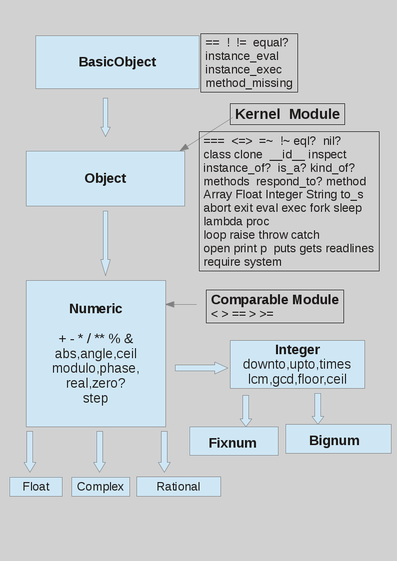# Numeric Classes

In the figure the class hierarchy of the numeric classes; some methods of the classes are listed in the figure.The classes for numbers are: Float, Complex, Integer, Rational; there are two subclass of Integer: Bignum and Fixnum; Bignum are numbers with an arbitrary number of digits; Fixnum are 8 bytes long integers, (but different platform can have different lengths); conversion between Bignum and Fixnum is automatically managed by Ruby: when a number is less than about 4E9 it's a Fixnum, otherwise it is a Bignum. Integer numbers can be built with the Integer method, converting its argument to an integer. Integers can also be expressed in hexadecimal, octal or binary form:

0x is the prefix for hexadecimal representations

0b is the prefix prefix for binary representation

0 is the prefix prefix for octal representation

Floats are double precision , always a digit is needed before and after the point: ".1" or "1." are not valid float numbers. "e" or "E" is before the (optional) exponent. Float numbers can be built with the Float method, converting its argument to a float number.

There are two special values for floats: "infinite" and "nan", to deal with float division by zero, but integer division by zero raises the exception: ZeroDivisionError

```0.0/0.0  => NaN
1.0/0.0  => Infinity

0/0      => ZeroDivisionError:  ....
```

Rational numbers are the ratio of two integers, used to express periodic numbers without a loss of precision. Complex numbers are printed as sum of a real and an imaginary part. Es.: (2+5i) .

Rational and complex have some specific methods and can be built with the "Rational and Complex methods:

``` Complex(1)           => (1+0i)
Complex(2, 3)        => (2+3i)
Complex(0.3)         => (0.3+0i)
Complex('0.3-0.5i')  => (0.3-0.5i)

Complex(2, 3).real  => 2           # real part
Complex(2, 3).imag  => 3           # imaginary part)
Complex(2, 3).conj  => (2-3i)

Complex(0,1).polar  => [1, 1.5707963267948966]  # modulus, phase
Complex(0,1).rect   => [0, 1]                   # Array with real and imaginary parts

a=Rational("1/2")
a=1.quo(5)            # division between integers that gives a rational number

Rational(1)      => (1/1)
Rational(2, 3)   => (2/3)
Rational(4, -6)  => (-2/3)
Rational('0.3')  => (3/10)
Rational('2/3')  => (2/3)
Rational('0.5')  => (1/2)

(Rational(3,2)).numerator     => 3
(Rational(3,2)).denominator   => 2
(Rational(3,2)).truncate      => 1
(Rational(3,2)).round         => 2
```

Underscores can be used inside numbers to separate digits:

```a=1_000_000 =>  1000000
b=2_000.0_1 =>  2000.01
```

## Methods for the Numeric Classes

All numerical operators are implemented as methods of the Numeric class: for this reason a function-like notation is legal for operators: "1.+(2)" can be used, and returns the number 3, as: "1+2".

The comparison operators: < ; > ; <= ; >=, == ; between? are implemented in the Comparable module; each numeric class implements in a different way only the operator: <=> (sometimes named: spaceship operator); the Comparable operators use the <=> operator of the specific class to give results. This mechanism is similar to the use virtual functions of the C++ language.

In the following table the most used methods of Numerics are included, also those which apply only to some subclasses of Numerics.

Operator Function Examples
** ; pow(x,y) power a**3
* / Multiplication,division a*b ; 3*2 => 6 ; 3/2=1
% Remainder 5/2. => 1.0
+ - Addition, subtraction a+b ; 2+5 => 7
<< >> bitwise shift 4<<1 => 8
& | ^ bitwise and, or, xor
<=> comparisons, returns: -1, 0 , 1 ; if less, equal greater
< > <= >= comparisons 4<1=> false
between? inclusion in a range 2.between?(1,4) => true
== != equal, not equal
zero? true if zero 3.2.zero? => false
eql? same value AND type 1.0.eql? 1 => false
equal? reference equality: if a is the same object as b: a.equal?(b) => true
abs ; magnitude Absolute value -1.abs => 1
abs2 square modulus -2.abs2 => 4
ceil minimum greater integer 1.2.ceil => 2 ; -1.2.ceil => -1
floor maximum lower integer 1.7.floor =>1 ; -1.2.floor =>-2
round rounds a number 1.4.round => 1 ; 1.5.round => 2
div integer division 5.2.div(2.0) => 2
divmod quotient and remainder 5.divmod(2) => Array :[2, 1]
fdiv float division 3.fdiv(2) => 1.5
quo division with maximum precision (Rational numbers if integer operands)

## Methods for Integers

 even? true if even 2.even? => true odd? true if odd 3.odd? => true next ; succ next integer 1.next => 2 pred previous integer 2.pred =>1 gcd greatest common denominator 10.gcd(15) => 5 lcm lowest common multiple 10.lcm 15 => 30

## Methods for Floats

 finite? true if finite (1.0/0.0).finite? => false infinite? test if infinite (-1.0/0.0).infinite? => -1 nan? test if not a number (0.0/0.0).nan? => true

## Conversion Methods

```**to_s**     # converts to string, for fixmum the argument is the base, es.:

16.to_s     => "16" ;
16.to_s(2)  => "10000" ;
16.to_s(16) => "10"

**to_i**     # converts to integer;

**to_f**     # converts to float;

**to_r**     # converts to rational
```

## Subscript Operator

The subscript operator "[]" can be used for Fixnum, and returns single bits of a number:

```2 => 0
2 => 1

3 => 1
3 => 1
3 => 0
```

## Precedence for operators

In the following some operators are listed, ordered by precedence (high to low precedence):

```[ ] [ ]=
**
! ~ + -      (unary operators)
* / %
+ -
>> <<
&
^ |
<= < > >=
<=> == === != =~ !~
&&
||
.. ...
?:
= %= /= -= += |= &= >>=
<<= *= &&= ||= **= ^=
not
or and
if unless while until
begin/end
```